#### 背景介绍，搜索引擎中query检索的主要问题：

1. query和document构词不太一样。例如query中为”how much tesla”，而文档中更多的是”price of tesla”。
2. 低频长尾网页的用户点击不可用，click modeling失效
3. 用户的一些提问表达或者口语化表达，如何进行意图理解
4. 时间维度的满足，例如”the safest cat”，返回”the safest cat is the 1998 toyota celica”可能不满足用户的需求。
5. 空间维度的满足，当用户搜索”restaurant”和”walmart”的时候，可能需要从搜索引擎中得到结果，按地理位置就近满足

#### 文章对于上述问题展开了叙述

1. 介绍了雅虎最work的排序函数，提出线下评估结果和在线搜索体验的优化点。
2. 探索如何将用户的点击信息从高频query转移到低频query。
3. 通过改写提高相关性，尤其是低频query的相关性。
4. 提出了时间空间(LBS)相关性的解决方案

#### 搜索引擎基础知识：

1. 搜索引擎的整体架构：
采用分治思路，文档通过url计算md5，根据md5进行分布式存储，每个机器视为一个节点，可以并行工作。当发生query查询时，第一轮粗排序，先在每个节点中找到所有匹配该query的文档，这个过程完成文档召回，这里会对文档进行一个简单的打分和去重，返回指定数目的候选文档，同时对这些选定文档进行query相关特征抽取。进入第二轮精排序使用一个核心排序函数(Core Ranking Function)打分，得到最佳的文档。
2. 所用到的特征如下：

1. Web graph, 通过www的链接情况进行文档质量打分，这里有大名鼎鼎的pagerank
2. Document statistics，一些文档相关的基础统计特征，如词频，词的数目等等
3. Document classifier，会用到一系列分类器，判断是否作弊文档，是否色情，文档语言，主题，质量和页面类型。
4. Query features， 词的数目，query频次和其中不同词的词频
5. Text match， 包括词之间的匹配，例如(query-doc.title), (query-doc.url), (query, doc.keywords), (query, doc.abstract)等等。这些特征可以进行组合形成新的特征，例如BM25. 以此判断文档和query的匹配程度
6. Topical matching， 语义层面的匹配。
7. Click， 利用用户的点击信息，预测包括prob of click, first click, last click, long dwell time click or only click。
8. Time， 对于强时效性需求的query，文档的时效性要求很高。
3. 排序结果的评价指标–搜索相关性
逐条对搜索结果进行分等级的打分, 分数有五档{Perfect: 5分, Excellent: 4分, Good: 3分, Fair: 2分, Bad: 1分}，假设文档在排序后的结果列表中位置为[1,N]，用如下DCG(Discounted Cumulative Gain)作为衡量指标：

$$DC{G_N} = \sum\limits_{i = 1}^N {{{{G_i}} \over {{{\log }_2}\left( {i + 1} \right)}}}$$

按：为了使得不同query下的结果容易比较，对DCG进行归一化得到$NDCG = {{DCG} \over {\max \_DCG}}$。作者为了评估时间相关性，引入时间的评价指标DCR和二者的综合指标RDDCG，后续内容会进一步介绍。
下面介绍下测试集，从一年log里面随机抽取出2000query，按query的频次又可以细分为高频，中频，低频三档。中频query基本上一年就几次点击，低频的话平均一年不到一次用户点击，用户点击信息对低频query基本无效。文章主要对中低频query相关性进行优化。

#### 用于排序的机器学习模型–gradient boosting decision trees

GBDT作为第二轮排序的打分模型，为了减少bad/fair url，作者选用(logistic loss)损失函数。如果将五档打分中的{perfect, excellent, good}视为正例(positive, +1)，{fair, bad}视为负例(negative, -1)，得到的损失函数为：
$$L\left( {y,F} \right) = \log \left( {1 + \exp \left( { - yF} \right)} \right),y \in \left\{ {1, - 1} \right\}$$

Logistic loss在2分类问题中，能有效降低不相关url在排序结果中头部占比，该loss除了用在分类，还能提供较为可靠的排序。和hinge loss不同，它能将positive/negative往positive/negative两个方向拉开，这样的话，如果一个url的相关性是perfect，那么它会距离决策平面远一些（按：放到下一篇进一步探讨）。

$$pseudo - residuals\left( x \right) = - {g_m}\left( {{x_i}} \right) \times scale\left( {label} \right)$$#### 基于上下文的重排序(Contextual Reranking)

1. 位置：url在精排序中的位置
2. 均值：精排序中各个特征的均值
3. 方差：精排序中各个特征的方差
4. 归一化特征：通过标准差和均值归一化后的特征
5. 主题模型特征：将top30url的主题分布累加起来作为query的topic分布，单独计算各url与query的topic分布的相似度
作者解释为什么使用第二轮的排序位置而不是模型打分作为重排序的输入，主要是实践结论，尽管模型打分包含更完整的信息，但是这个值很容易发生波动。（按：原文Though the core-ranking phase score is more informative than the rank, its value can drift as the index refreshes, degrading third phase performance索引这里猜测是建库的索引，只是query-url应该不受影响，费解）。实验结果显示在table2，在部署的时候，精排序的模型打分可以并行进行，但重排序只能在一个机器节点中完成，Yahoo在重排序中用了30-50组原始特征。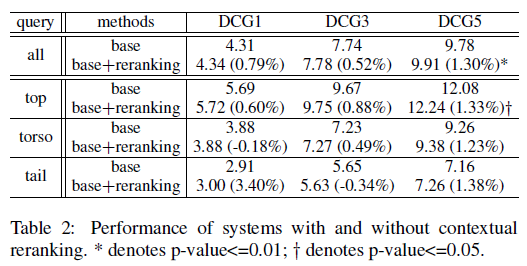#### 语义匹配特征

$$DV_i^n = {1 \over {{{\left\| {\sum\nolimits_{i = 1}^{\left| Q \right|} {{C_{ij}} \cdot QV_i^n} } \right\|}_2}}}\sum\nolimits_{i = 1}^{\left| Q \right|} {{C_{i,j}} \cdot QV_i^n}$$

$$QV_i^{n + 1} = {1 \over {{{\left\| {\sum\nolimits_{j = 1}^{\left| D \right|} {{C_{ij}} \cdot DV_j^n} } \right\|}_2}}}\sum\nolimits_{j = 1}^{\left| D \right|} {{C_{i,j}} \cdot DV_j^n}$$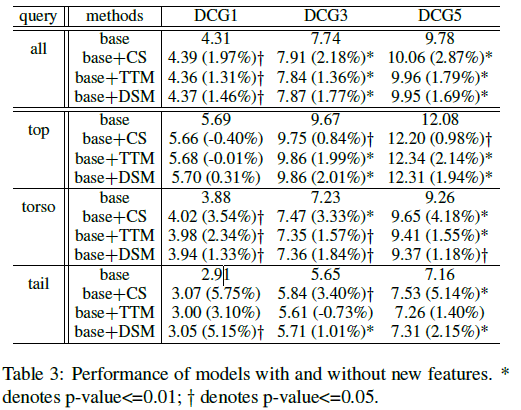CS特征无法用在未出现在点击日志的query和document之间。统计机器翻译将query翻译到document，通过机器翻译带来一系列特征，称为”Translated Text Matching(TTM)”.

CS特征和TTM部分解决了中长尾query的文本和用户行为信息不足，CS用来平滑点击信息并去除噪音点击，而TTM是query改写的一个应用。这些方法停留在词级别。我们需要进行语意级别的匹配，这里用DSSM进行学习。(按：DSSM可以看高剑锋老师的报告，在度学堂有)，记query集合$Q$和其候选document集合${D_ \pm }$。得到query和document的向量表达之后用如下softmax函数计算似然：
$$L\left( {{D_ + }|Q} \right) = {{\exp \left( { - \cos \left( {{V_Q},{V_{D + }}} \right)} \right)} \over {\sum\nolimits_{D \pm } {\exp \left( { - \cos \left( {{V_Q},{V_{D \pm }}} \right)} \right)} }}$$

#### query改写

$${q_w} = \mathop {\arg \max }\limits_{{q_c}} \sum\nolimits_{i = 1}^m {{\lambda _i}{h_i}\left( {{q_c},q} \right)}$$

1. query feature functions: query $q$中词数目，停用词数目，语言模型打分，query频次，词的平均长度
2. rewrite query feature functions：改写后query $q_c$中词数目，停用词数目，语言模型打分，query频次，词的平均长度
3. pair feature functions：$h_{11}$：在query-url连接图中$q$和$q_c$的jaccard相似度。$h_{12}$原始query $q$与改写后$q_c$的频次的差、$h_{13}$二者词级别的cos相似度、不相同的词个数、相同词的个数、语言模型打分的差，停用词数目的差、平均词长度的差。
实践中$h_{11}$、$h_{12}$、$h_{13}$是三个最有影响的特征。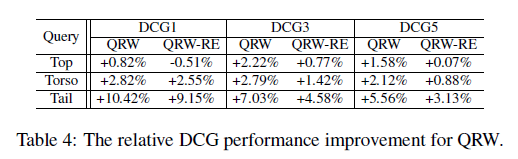#### 组合实验

“base”：仅LogistcRank
“GBrank”：为传统的GBDT用于排序。
“base+feat”： LogistcRank+语意特征(CS, TTM, DSM)。
“base+all”： LogistcRank+语意特征(CS, TTM, DSM)+改写，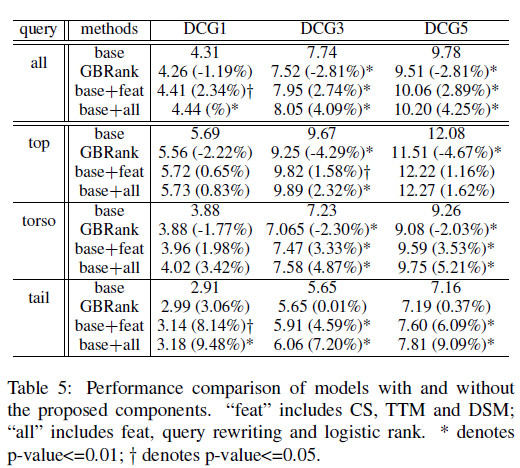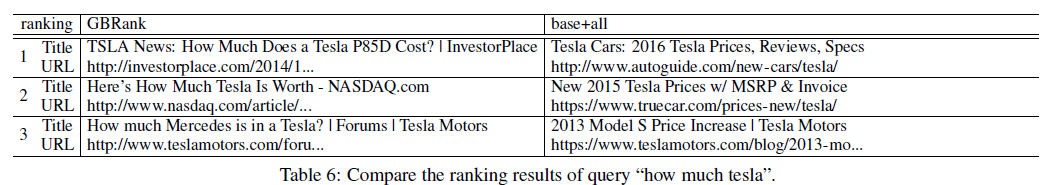#### 时效性满足排序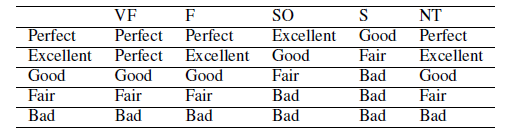$$f\left( x \right) = \left\{ {\matrix{ {{f_{relative}}\left( x \right) + {r_{fresh}}\left( x \right)\;\;if\;{c_{ts}}\left( x \right) > 0} \cr {{f_{relative}}\left( x \right)\;\;\;\;\;\;\;\;\;\;elsewise.} \cr } } \right.$$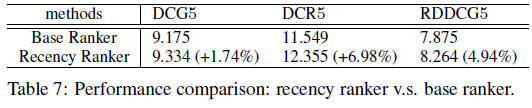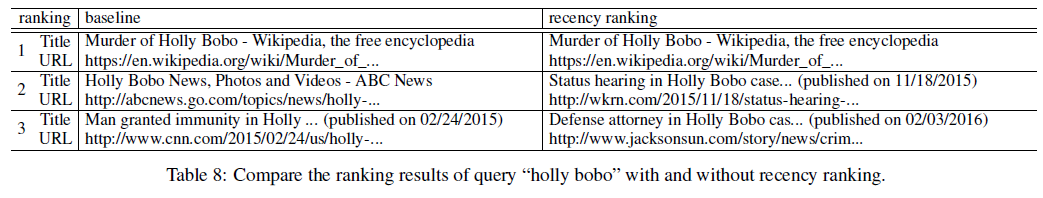#### 空间位置满足排序

$$f\left( x \right) = {f_{relative}}\left( x \right) + w{1 \over {1 + {e^{\alpha {f_{relative}}\left( x \right) + \beta }}}}\hat d\left( {QUERY,URL} \right)$$
PS.作者论，改进后的logistic函数根据$f_{relative}( x )$控制地理位置相关性打分。

pair-wise训练，其中$P = \left\{ {\left( {{p_i},{p_j}} \right)|\;{p_i} > {p_j}} \right\}$为一个query下的偏序关系的url-pair。${p_i} > {p_j}$表示给定query下${p_i}$比${p_j}$结果更好，使用随机梯度下降(SGD)可以解之。
（按，感觉这个打分的设计有点复杂，可以再考虑简化下）。

1.gbrank中怎么加入pointwise信息，后续了解
2.丝路搜索中看holly bobo，百度对英文时效性query是否有进行类似的调整。也可能是时效性需求识别未召回。
3.百度在中文检索中进行的探索深度和复杂程度不局限于作者提的这些方法和特性，例如色情类query屏蔽（国际化搜索则称色情query满足）、图片需求类query、用户个性化等等。wise端的场景更加丰富和具有挑战。

#### 原文及部分引文：

Yin, Dawei, Hu, Yuening, Tang, Jiliang, et al. Ranking Relevance in Yahoo Search[C] ACM SIGKDD International Conference on Knowledge Discovery and Data Mining. ACM, 2016.
C. J. C. Burges. From RankNet to LambdaRank to LambdaMART: An overview. Technical report, Microsoft Research, 2010.
P.-S. Huang, X. He, J. Gao, L. Deng, A. Acero, and L. Heck. Learning deep structured semantic models for web search using clickthrough data. In CIKM ’13.
K. Zhou, X. Li, and H. Zha. Collaborative ranking: Improving the
relevance for tail queries. In CIKM ’12.